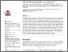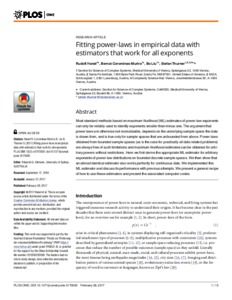# Fitting power-laws in empirical data with estimators that work for all exponents

Altmann, E.G., Hanel, R., Corominas-Murtra, B., Liu, B., & Thurner, S. (2017). Fitting power-laws in empirical data with estimators that work for all exponents. PLOS ONE 12 (2) e0170920. 10.1371/journal.pone.0170920.Preview
Text
journal.pone.0170920.pdf - Published Version

## Abstract

Most standard methods based on maximum likelihood (ML) estimates of power-law exponents can only be reliably used to identify exponents smaller than minus one. The argument that power laws are otherwise not normalizable, depends on the underlying sample space the data is drawn from, and is true only for sample spaces that are unbounded from above. Power-laws obtained from bounded sample spaces (as is the case for practically all data related problems) are always free of such limitations and maximum likelihood estimates can be obtained for arbitrary powers without restrictions. Here we first derive the appropriate ML estimator for arbitrary exponents of power-law distributions on bounded discrete sample spaces. We then show that an almost identical estimator also works perfectly for continuous data. We implemented this ML estimator and discuss its performance with previous attempts. We present a general recipe of how to use these estimators and present the associated computer codes.

Item Type: Article Advanced Systems Analysis (ASA) Romeo Molina 01 Mar 2017 13:35 27 Aug 2021 17:41 http://pure.iiasa.ac.at/14397View Item